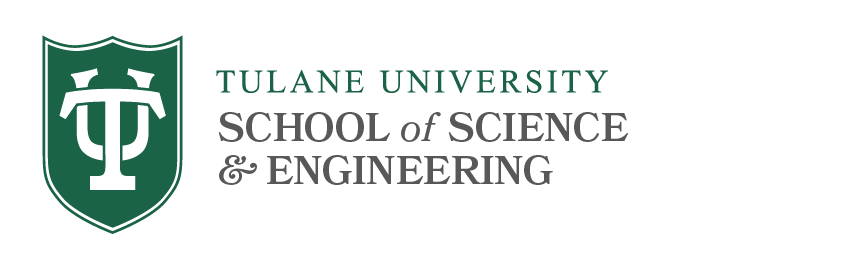# PDE Qualifying Exams

## Syllabus Topics

The following topics & references will prepare you for the exam.

1. Classical weak and strong maximum principles for 2nd order elliptic and parabolic equations, Hopf boundary point lemma, and their applications. References: pp. 326-334 of ; pp. 51-80, pp. 159-177 of ; pp. 31-37 of .
2. Sobolev spaces, weak derivatives, approximation, density theorem, traces,Sobolev inequalities, Kondrachov compact imbedding. References: pp. 239-274 of ; pp.144-158, pp. 167-171 of .
3. L² theory for second order elliptic equations, existence via Lax-Milgram Theorem, Fredholm alternative, L² estimates/regularity, eigenvalue problem and eigenexpansion. References: pp. 293-326, pp. 334-340 of ; pp. 181-188, pp. 212-214 of .
4. L² theory for second order parabolic and hyperbolic equations, existence via Galerkin method (the special case being the eigen-expansion method), uniqueness and regularity via energy method. References: pp. 349-367, 377-393 of .
5. A brief introduction to elliptic regularity theory: the Lp and Schauder estimates. For this part, only need to know how to apply the theory. References: Theorems 6.2, 6.6, 9.13, 9.14 and 9.19 of .
6. Nonlinear elliptic equations, variational methods, fixed point method, method of upper and lower solutions. References: pp. 498-511 of , pp. 385-398 of .
7. Conservation laws, Rankine-Hugoniot jump condition, uniqueness issue, entropy condition, Riemann problem. References: pp. 136-157 of .

References
1. Partial Differential Equations, by L. Evans, Graduate Studies in Math, Vol 19, AMS.
2. Maximum Principles in Differential Equations, by M. Protter and H. Weinberger, Prentice-Hall.
3. Elliptic Partial Differential Equations of Second Order, 1998 edition, by D. Gilbarg and N. Trudinger, Springer.
4. Partial Differential Equations, 2nd edition, by R. McOwen, Prentice-Hall.